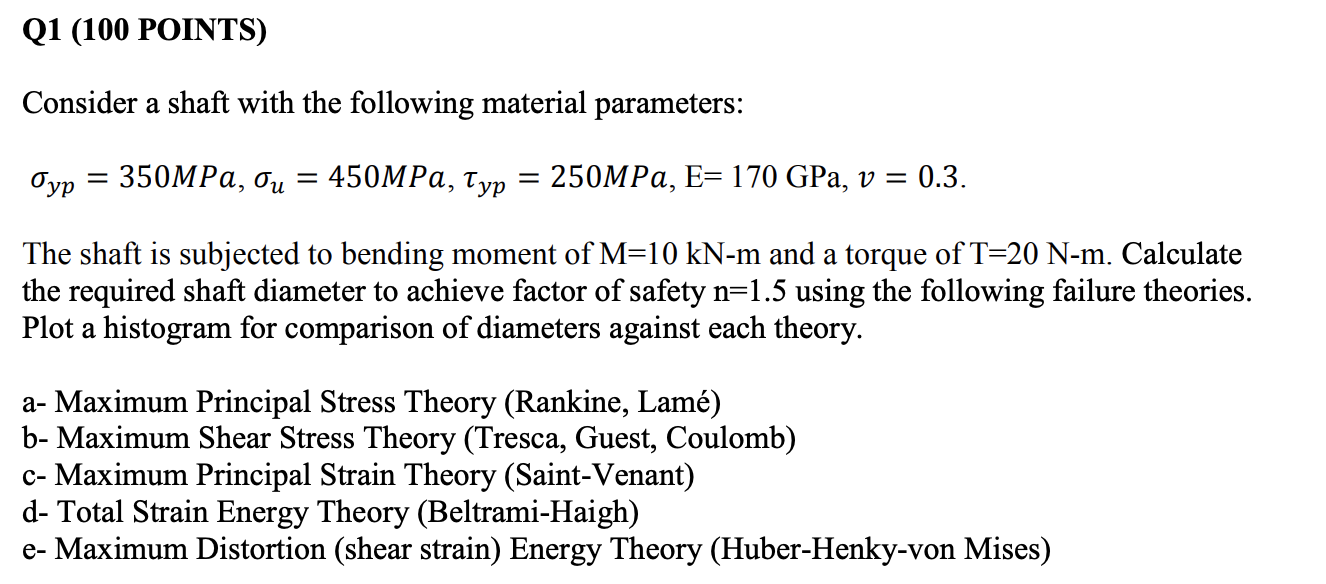Home / Answered Questions / Other / q1-100-points-consider-a-shaft-with-the-following-material-parameters-oyp-350mpa-ou-450mpa-typ-250mp-aw186

# (Solved): Q1 (100 POINTS) Consider A Shaft With The Following Material Parameters: Oyp = 350MPa, Ou = 450MPa, ...Q1 (100 POINTS) Consider a shaft with the following material parameters: Oyp = 350MPa, Ou = 450MPa, Typ = 250MPa, E= 170 GPa, v = 0.3. The shaft is subjected to bending moment of M=10 kN-m and a torque of T=20 N-m. Calculate the required shaft diameter to achieve factor of safety n=1.5 using the following failure theories. Plot a histogram for comparison of diameters against each theory. a- Maximum Principal Stress Theory (Rankine, LamÃ©) b- Maximum Shear Stress Theory (Tresca, Guest, Coulomb) c- Maximum Principal Strain Theory (Saint-Venant) d- Total Strain Energy Theory (Beltrami-Haigh) e- Maximum Distortion (shear strain) Energy Theory (Huber-Henky-von Mises)

We have an Answer from Expert